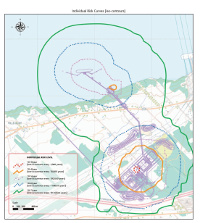# Risk modelling and quantification The probabilistic approach to safety

## OverviewRather than using point values to represent random quantities, as in deterministic approaches, probabilistic approaches to risk assessment represent each random quantity by a probability distribution. Analysis results are presented as probability distributions, or as selected quantiles of a probability distribution.

The probabilistic approach to risk analysis estimates risk as a function of:

• the severity — or magnitude — of each consequence
• the likelihood (probability) of the occurrence of each consequence

In the safety domain, the consequences and types of events assessed are generally adverse (they represent losses, that we try to avoid). In other areas such as finance, events and their consequences may be either positive (profits) or negative (losses).

Consequences are expressed quantatively (for instance, the number of people potentially injured) and their likelihoods of occurrence are expressed as probabilities.

## Other resources

We recommend the following sources of further information on this topic:

Published: